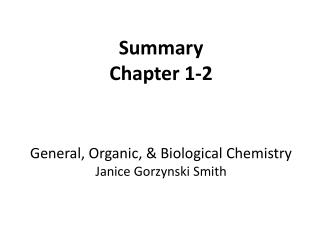DownloadDownload PresentationSummary Chapter 1-2 General, Organic, & Biological Chemistry Janice Gorzynski Smith

# Summary Chapter 1-2 General, Organic, & Biological Chemistry Janice Gorzynski Smith

Download Presentation## Summary Chapter 1-2 General, Organic, & Biological Chemistry Janice Gorzynski Smith

- - - - - - - - - - - - - - - - - - - - - - - - - - - E N D - - - - - - - - - - - - - - - - - - - - - - - - - - -
##### Presentation Transcript

1. Summary Chapter 1-2 General, Organic, & Biological Chemistry Janice GorzynskiSmith

2. CH 1-2 Concepts to be Familiar With • Classification of matter: pure substances & mixtures • Homogeneous vs Heterogeneous • Distinguish the difference between chemical and physical properties & changes • We represent uncertainty with significant figures • You do not need to memorize Sig Fig rules • Scientific Notation • Conversions within the metric system and non metric units • Temperature conversions • Density & Specific gravity • Familiarity with how compounds will be drawn • Molecular formulas • Structure of an atom: protons, neutrons, electrons • Atomic number, isotope mass number, atomic weight • Navigate the periodic table: properties shared within a group, trends, metals/metalloids/nonmetals • Determine valance electrons & draw electron dot representations • Ionization Energy & Atomic Size

3. Conversions & Equations To Memorize Unit Conversions For metric units (m, kg, s, K, mole): mega (M) 106 kilo (k) 103 centi (c) 10-2 milli (m) 10-3 micro (μ) 10-6 nano (n) 10-9 Pico (p) 10-12 Time conversions: dhrms 1 mL = 1 cm3 T(kelvin) = T(°C) + 273.15 Equations Density = mass / Volume d = m/V dH2O = 1 g/mL = 1 g/cm3 Specific Gravity = density substance / density of water y x 10x Exponent: Any positive or negative whole number. Coefficient: A number between 1 and 10.

4. Elements & Molecules X = Element symbol (ie O = oxygen) A = Isotope Mass Number = # protons + # neutrons Z = Atomic Number = # protons 6 C 12.01 atomic number Elements on the Periodic Table element symbol atomic weight (amu) = weighted average of atomic weight of isotopes • Molecular Formula: AxByEx: CH3O Drawing Molecules: Methane CH4

5. Properties of Groups

6. Properties of Metals, Nonmetals, Metalloids

7. Valence Electrons Example: Determine the valence electrons of Selenium (Se): Find Se on the periodic table Focus on just the row (period) Se is in Count the number of electrons in the s and p orbitals (ie, count to Se from the left side of the row) Count the number of elements in the row (period) that lead up to the element (Se). Remember, do NOT count the transition metals, lacthanides, and actinides. 1 2 X X X X X X X X X X 3 4 5 6 Electron Dot Symbols: Represent the valence electrons by drawing them around the element symbol for Selenium. Se

8. Periodic Trends Size INCREASING Ionization Energy INCREASING